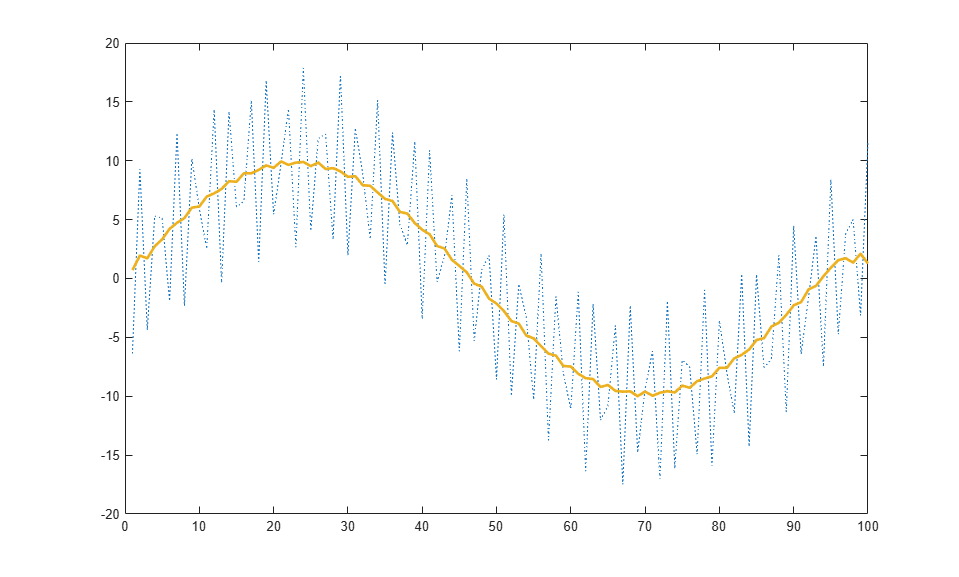# Chart Class with Custom Property Display

This example shows how to define a class of charts with a custom property display that lists only a subset of the properties. The following code demonstrates how to overload the `getPropertyGroups` method of the `matlab.mixin.CustomDisplay` class. The example also demonstrates the basic coding pattern for charts that derive from the `matlab.graphics.chartcontainer.ChartContainer` base class. You can use this example to become familiar with the coding techniques of chart development, or as the basis for a class you plan to develop.

To define the class, copy the following code into the editor and save it with the name `SmoothPlotCustomDisplay.m` in a writable folder.

```classdef SmoothPlotCustomDisplay < matlab.graphics.chartcontainer.ChartContainer properties XData (1,:) double = NaN YData (1,:) double = NaN SmoothColor (1,3) double {mustBeGreaterThanOrEqual(SmoothColor,0),... mustBeLessThanOrEqual(SmoothColor,1)} = [0.9290 0.6940 0.1250] SmoothWidth (1,1) double = 2 end properties(Access = private,Transient,NonCopyable) OriginalLine (1,1) matlab.graphics.chart.primitive.Line SmoothLine (1,1) matlab.graphics.chart.primitive.Line end methods(Access = protected) function setup(obj) % Get the axes ax = getAxes(obj); % Create the original and smooth lines obj.OriginalLine = plot(ax,NaN,NaN,'LineStyle',':'); hold(ax,'on') obj.SmoothLine = plot(ax,NaN,NaN,'LineStyle','-',... 'Color',[0.9290 0.6940 0.1250],'LineWidth',2); hold(ax,'off') end function update(obj) % Update line data obj.OriginalLine.XData = obj.XData; obj.OriginalLine.YData = obj.YData; obj.SmoothLine.XData = obj.XData; obj.SmoothLine.YData = createSmoothData(obj); % Update line color and width obj.SmoothLine.Color = obj.SmoothColor; obj.SmoothLine.LineWidth = obj.SmoothWidth; end function propgrp = getPropertyGroups(obj) if ~isscalar(obj) % List for array of objects propgrp = getPropertyGroups@matlab.mixin.CustomDisplay(obj); else % List for scalar object propList = {'SmoothColor','XData','YData'}; propgrp = matlab.mixin.util.PropertyGroup(propList); end end function sm = createSmoothData(obj) % Calculate smoothed data v = ones(1,10)*0.1; sm = conv(obj.YData,v,'same'); end end end ```

After saving the class file, you can create an instance of the chart. Omit the semicolon when you create the chart to see the customized display.

```x = 1:1:100; y = 10*sin(x/15)+8*sin(10*x+0.5); c = SmoothPlotCustomDisplay('XData',x,'YData',y) ```
```c = SmoothPlotCustomDisplay with properties: SmoothColor: [0.9290 0.6940 0.1250] XData: [1x100 double] YData: [1x100 double] Use GET to show all properties ```